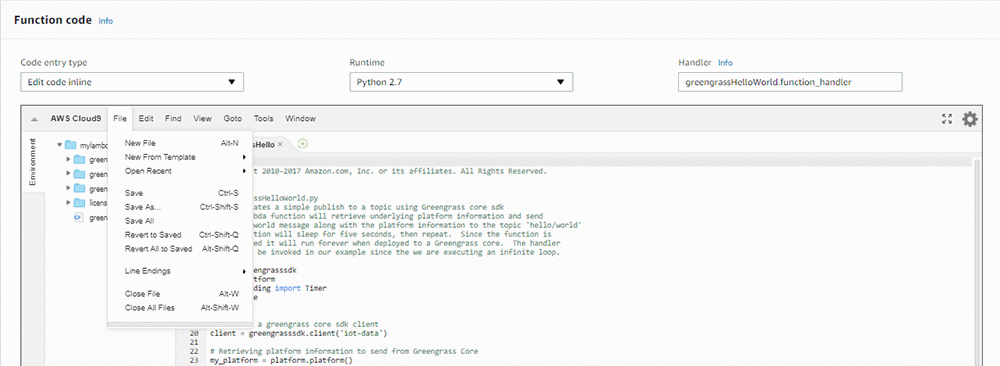# AWS DeepLens Lambda 函数与新 Model Optimizer 详解

AWS DeepLens 使用深度神经网络计算库 (Cl-DNN) 来访问推断 GPU。模型必须转换为 Cl-DNN 格式后才能在 AWS DeepLens 上运行。根据具体模型的大小，只需输入如下一行代码，Model Optimizer 可在 2-10 秒内完成这一转换：

``mo.optimize(model_name,input_width,input_height)``

1. 选择创建函数。对此函数进行自定义，以运行深度学习模型的推断。
2. 选择蓝图
3. 搜索 greengrass-hello-world 蓝图。
4. Lambda 函数名必须与模型名相同。
5. 选择现有的 IAM 角色：AWSDeepLensLambdaRole。 此决策必须在注册过程中事先创建。
6. 选择创建函数
7. 函数代码中的句柄必须是 greengrassHelloWorld.function_handler
8. 在 GreengrassHello 文件中，应清除所有代码。您将在此文件中写入推断 Lambda 函数的代码。

``````import os
import greengrasssdk
import awscam
import mo
import cv2

``client = greengrasssdk.client('iot-data')``

``iot_topic = '\$aws/things/{}/infer'.format(os.environ['AWS_IOT_THING_NAME'])``

``````jpeg = None
Write_To_FIFO = True``````

``````class FIFO_Thread(Thread):
def __init__(self):
''' Constructor. '''

def run(self):
fifo_path = "/tmp/results.mjpeg"
if not os.path.exists(fifo_path):
os.mkfifo(fifo_path)
f = open(fifo_path,'w')
while Write_To_FIFO:
try:
f.write(jpeg.tobytes())
except IOError as e:
continue
``````

``````def greengrass_infinite_infer_run():
input_width = 224
input_height = 224``````

` input_width``input_height` 参数定义了模型的输入参数。如要执行推断，模型要求帧的大小相同。对于您部署到 AWS DeepLens 的模型，您可以自定义这些参数。

``model_name = 'squeezenet_v1.1'``

``error, model_path = mo.optimize(model_name,input_width,input_height)``

``model = awscam.Model(model_path, {"GPU": 1})``

``client.publish(topic=iot_topic, payload="Model loaded")``

``model_type = "classification"``

1. 在函数代码部分，选择文件。然后选择新建文件2. 将文本文件中的数据粘贴到您刚刚创建的新文件中
3. 选择文件，然后选择保存
4. 输入文件名 sysnet.txt，然后选择保存

``````with open('sysnet.txt', 'r') as f:
labels = [l.rstrip() for l in f]``````

``topk = 5``

``````results_thread = FIFO_Thread()

`Inference is starting` 消息发布到 AWS IoT 控制台。在接下来的几行中，您将编写推断循环的代码。

``client.publish(topic=iot_topic, payload="Inference is starting")``

``````while True:
ret, frame = awscam.getLastFrame()
if ret == False:
raise Exception("Failed to get frame from the stream")

frame_resize = cv2.resize(frame, (input_width, input_height))``````

``infer_output = model.doInference(frame_resize)``

``parsed_results = model.parseResult(model_type, infer_output)``

``top_k = parsed_results[model_type][0:topk]``

``````msg = "{"
prob_num = 0
for obj in top_k:
if prob_num == topk-1:
msg += '"{}": {:.2f}'.format(labels[obj["label"]], obj["prob"])
else:
msg += '"{}": {:.2f},'.format(labels[obj["label"]], obj["prob"])
prob_num += 1
msg += "}"
``````

``client.publish(topic=iot_topic, payload=msg)``

``cv2.putText(frame, labels[top_k["label"]], (0,22), cv2.FONT_HERSHEY_SIMPLEX, 1, (255, 165, 20), 4)``

``````global jpeg
ret,jpeg = cv2.imencode('.jpg', frame)
``````

``````except Exception as e:
msg = "Lambda failed: " + str(e)

``greengrass_infinite_infer_run()``

### 作者简介Jyothi Nookula 是一名 AWS DeepLens 高级产品经理。她热爱创造客户满意的产品，在空余时间，她热爱绘画和主持自己作品的慈善义卖。Eddie Calleja 是一名 AWS 深度学习软件开发工程师。他是 DeepLens 设备的开发人员之一。他以前是一名物理学家，他经常利用空闲时间思考如何运用人工智能技术来解决现代物理学问题。